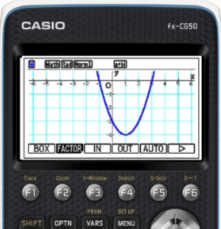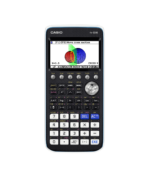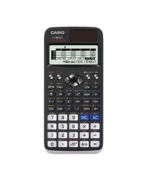Resources Archive - Casio Calculators

# Resources

Welcome to the Casio Resources Centre where you can find, view, save and download over 200 resources, created by teachers for teachers. Below you will find our most popular collections. Simply use the pre-defined collection buttons or filter facility to source the resources you need. Create your own account so that you can save and build your own resources list.

Filter

### Equations21 Resources

View AllHow to Video
fx-CG50 A-Level GCSE IB
Quick Skill: Solving Linear Equations

How to solve a linear equation using the numerical SolveN function....How to Video
fx-CG50 IB A-Level GCSE
Quick Skill: Simultaneous Equations – Using Equation Solutions

How to extract the solutions to a system of linear simultaneous equations found ...How to Video
fx-CG50 A-Level GCSE IB
Quick Skill: Polynomials – Using Equation Results

How to extract a polynomial's solutions found using the Equation app. You will n...How to Video
fx-CG50 GCSE IB A-Level
Simultaneous Equations – Solving Numerically

How to use Equation to solve simultaneous linear equations in two variables nume...

### Functions50 Resources

View AllHow to Video
fx-CG50 A-Level GCSE IB
Quick Skill: Calculations Using Coordinate Values

How to extract coordinate values from points of interest in Graph to perform ope...How to Video
fx-CG50 A-Level GCSE IB
Quick Skill: Graphing – Storing and Recalling Functions

How to use Graph Memory to store functions and settings for later recall. You wi...How to Video
fx-CG50 A-Level GCSE IB
Quick Skill: Table – Extracting Values

How to extract either a single column or a complete set of table values. You wil...How to Video
fx-CG50 A-Level GCSE IB
Quick Skill: Graphing – Drawing a Graph and Table

How to draw the graph of a function and create a table of values from the graph....

### Trigonometry11 Resources

View AllHow to Video
fx-CG50 A-Level GCSE IB
Quick Skill: Calculations Using Coordinate Values

How to extract coordinate values from points of interest in Graph to perform ope...How to Video
fx-CG50 A-Level GCSE IB
Quick Skill: Graphing – Storing and Recalling Functions

How to use Graph Memory to store functions and settings for later recall. You wi...How to Video
fx-CG50 A-Level GCSE IB
Quick Skill: Table – Extracting Values

How to extract either a single column or a complete set of table values. You wil...How to Video
fx-CG50 A-Level GCSE IB
Quick Skill: Graphing – Drawing a Graph and Table

How to draw the graph of a function and create a table of values from the graph....

### Sequences and Series5 Resources

View AllHow to Video
fx-CG50 A-Level GCSE IB
Quick Skill: Sequences – Generating Terms

How to generate a list of terms of a sequence based on a function and then sum t...How to Video
fx-CG50 A-Level IB
Quick Skill: Sequences – Summation

How to use sigma summation of the terms of a sequence....How to Leaflet
fx-CG50 A-Level IB
Recursion Quick Start fx-CG50

How to create a table of sequence values on the fx-CG50. Includes plotting a rec...How to Video
fx-CG50 A-Level
Sequences – Recursion and Equations

How to iterate using ANS to generate terms in arithmetic and geometric sequences...

### Statistical Data17 Resources

View AllHow to Video
fx-CG50 A-Level GCSE IB
Quick Skill: Quartiles – Using Summary Values

How to extract the quartile values of single-variable data found using the Stati...How to Video
fx-CG50 IB A-Level GCSE
Quick Skill: Statistics – Using Summary Values

How to extract the summary statistic values of single-variable data found using ...How to Video
fx-CG50 A-Level GCSE IB
Statistics – Managing Lists

How to enter and manipulate data in Lists. Set frequency tables. Sort entries a...How to Video
fx-CG50 GCSE IB A-Level
Single Variable Statistics

How to calculate single variable statistics such as mean, standard deviation and...

### Mechanics3 Resources

View AllHow to Video
fx-CG50 A-Level GCSE IB
Quick Skill: Solving Equations with more than 1 Variable

How to use Solver to solve equations with more than one variable including SUVAT...How to Video
fx-CG50 A-Level IB
Calculus in Mechanics – Derivatives

How to analyse the position equation of a particle to find average, instant and ...How to Video
fx-CG50 IB A-Level
Calculus in Mechanics – Derivative Functions and Integration

How to plot and analyse the relationship between the derivative functions of the...

### Financial1 Resources

View AllHow to Leaflet
fx-CG50 IB
Financial Quick Start fx-CG50

How to calculate compound interest on the fx-CG50....

### Coordinate Geometry22 Resources

View AllHow to Video
fx-CG50 A-Level
Tangents and Normals – Parametric Functions

How to sketch the tangent and normal to a curve in parametric form. Includes fin...How to Video
fx-CG50 A-Level IB
Tangents and Normals – Cartesian Functions

How to sketch the tangent and normal to a curve in Cartesian form. Includes find...How to Video
fx-CG50 A-Level
Simultaneous Equations – Solving Harder Equations Graphically

How to solve a simultaneous linear and quadratic equation in both x and y graphi...

### Vectors and Matrices5 Resources

View AllHow to Video
fx-CG50 Further Maths IB
Quick Skill: Matrices – Inverses and Powers

How to find the inverse of a matrix and raise a matrix to a power. You will need...How to Leaflet
fx-CG50 Further Maths
Matrices and Vectors – Calculations (Worksheet)

Worksheet to accompany the video on how to define dimensions and store matrices ...How to Leaflet
fx-991EX Further Maths
Matrix Quick Start fx-991EX

How to define and enter a matrix. Perform calculations and find the determinant....How to Leaflet
fx-991EX Further Maths
Vector Quick Start fx-991EX

How to define dimensions and store vectors. Perform calculations such as subtrac...

### Probability Distributions22 Resources

View AllHow to Video
fx-CG50 Further Maths
Quick Skill: Poisson and Geometric Distributions – Finding X-Values

How to calculate X-values and critical values when using the Distribution app. Y...How to Video
fx-CG50 Further Maths
Quick Skill: Geometric and Poisson Distributions – Probabilities

How to calculate Geometric and Poisson probabilities. You will need to know how ...How to Video
fx-CG50 A-Level IB
Quick Skill: Normal Distribution – Extracting X-Values

How to extract X-values calculated using the Distribution app. You will need to ...How to Video
fx-CG50 A-Level IB
Quick Skill: Probability Distributions – Using Probability Results

How to extract probabilities calculated using the Distribution app. You will nee...

### Calculus13 Resources

View AllHow to Video
fx-CG50 A-Level IB
Quick Skill: Calculations Using Function Notation

How to use function notation to perform operations in the Run-Matrix app. You wi...How to Video
fx-CG50 A-Level IB
Calculus in Mechanics – Derivatives

How to analyse the position equation of a particle to find average, instant and ...How to Video
fx-CG50 IB A-Level
Calculus in Mechanics – Derivative Functions and Integration

How to plot and analyse the relationship between the derivative functions of the...How to Video
fx-CG50 A-Level
Tangents and Normals – Parametric Functions

How to sketch the tangent and normal to a curve in parametric form. Includes fin...

### Calculation16 Resources

View AllHow to Video
fx-CG50 A-Level GCSE IB
Quick Skill: Solving Equations with more than 1 Variable

How to use Solver to solve equations with more than one variable including SUVAT...How to Video
fx-CG50 A-Level Further Maths
Quick Skill: Memory – Using Run-Matrix

How to store values using Alpha memory and use them in later calculations....How to Video
GCSE A-Level
Table of Values fx-991EX

How to create a table of values of a function with incremental steps. Extend th...How to Video
fx-CG50 GCSE IB A-Level
Table – Calculating Values

How to calculate a table of values for a function. Change the step interval and ...